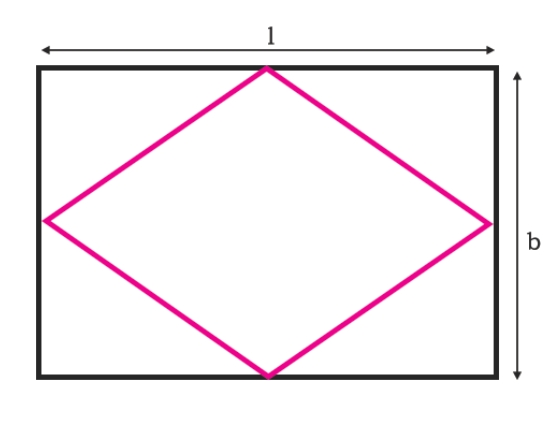# Area of the biggest possible rhombus that can be inscribed in a rectangle in C Program?

CServer Side ProgrammingProgramming

Here we will see one problem, where one rectangle is given. We have to find the area of largest rhombus that can be inscribed in the rectangle. The diagram will be look like below −The length of the rectangle is ‘l’ and breadth is ‘b’ So the area of the rhombus is −

## Source Code

#include <iostream>
#include <cmath>
using namespace std;
float area(float l, float b) {
if (l < 0 || b < 0) //if the values are negative it is invalid
return -1;
float area = (l*b) /2;
return area;
}
int main() {
float l = 20.0, b = 7;
cout << "Area : " << area(l, b);
}

## Output

Area : 70